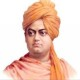# Reasoning: Machine Input-Output Questions Set 22 (SBI PO Pattern)

Directions (1-5): A string of numbers is given as input. The further steps given are obtained by applying certain logic. Numbers of step II have been obtained by using at least 1 digit of each number in step I. Each step is a resultant of previous step only.Input:1. What is the average of numbers obtained in step 2?
A) 23
B) 26
C) 25
D) 35
E) Other than options given
Option A
Solution:
For given example:For given input:::
Step I: 89……78…..86
Step II:     23……23
Step III:     1.5……..1.5
Step IV:           0
2. What is the second largest number obtained in any step of given input?
A) 89
B) 86
C) 78
D) 23
E) 45
Option B
3. Find the difference between sum of numbers obtained in 1st step and sum of numbers obtained in all other steps.
A) 211
B) 221
C) 225
D) 204
E) 215
Option D
Solution:
Required difference = (89+78+86) – (23+23+1.5+1.5+0)
4. What is the difference between the largest and the third smallest numbers obtained in any steps?
A) 84
B) 70
C) 66
D) 61
E) 58
Option C
Solution:
Required difference = 89 – 23
5. What is the average of numbers obtained in last 2 steps?
A) Other than given in options
B) 0.5
C) 2
D) 1.5
E) 1
Option E
Solution:
Required average =(1.5+1.5+0)/3 = 1

Directions (6-10): Consider the following steps for given input and read the instructions to reach to the last step.Instructions:
Step-I: Interchange the alphabets in input as arrows mentioned

Step-II: (i) If both letters are consonant and number is less than 6, then consonants change to previous letter in English alphabetical series.
(ii) If there is one vowel and one consonant, then add 4 to the number.
(iii) It there is single consonant, then consonant changes to next letter in English alphabetical series.

Step-III: has been derived using a special pattern taking similar (but not exactly) patterns of both step I and step II.1. What is the sum of all numbers in step II of given input?
A) 42
B) 40
C) 33
D) 36
E) 43
Option A
Solution:

In step III, we see that same number of alphabets are at same position, A changes to B, UM to TM, T to U, so if we take same pattern of step I, i.e. keeping letters constant and exchanging, we get the second figure as in below::Now try some application in this second figure as were given for step-II:
We see when there is single letter, letter change to next letter.
When both are consonants, then number is (number-2) e.g QF6 –> QF4
And when one is vowel and other consonant, then vowel changes to previous letter and number to (number+1).  like in BI8 B is B, I -> H and 8->(8+1)9
For given input:2. In last step, which of the following letter/s occur more than 1 time?
A) C
B) P
C) N
D) Both T and F
E) Both N and T
Option E
3. Which of the following represents the second element in 3rd row in step III?
A) L4
B) M4
C) L6
D) N4
E) M3
Option B
4. What is the addition of numbers which are with L and LR in step II?
A) 7
B) 5
C) 6
D) 3
E) 4
Option A
5. What is the addition of numbers which are with N, BT and TC in step III?
A) 17
B) 13
C) 16
D) 15
E) 19
Option D

## 23 Thoughts to “Reasoning: Machine Input-Output Questions Set 22 (SBI PO Pattern)”

1.naruto

thanx

2.Player

in q(6-10) what is the logic of step 3rd ???/

1.Shubhra

In this. 2 steps are mixed i.e.I and II.
Check figure 1 in solution part.

1.-HOPE-

is mai later to samajh me aa raha h but nunber kese arrang hua h

1.Shubhra

Step 1 me aane k liye jese letters ko change kiye bina numbers ko interchange kiya tha.
Vse hi phle step 2 me vesa karo. Interchange numbers with respect to arrow s.
Now derive rules from this point to step 3

1.-HOPE-

lekin BT6 -> BT5, LR1-> LR6. UC8 ->TC2, IN7-> HN7 me number kese arrang ho raha hai

2.Richard Parker

Mam please explain step 3 in q2, how does BT6->BT5 why not BT4 ?also UC8 -> TC2 and not TC9?

3.Face The Real World\$\$

In step 3 F8..how didn’t get it

4.thinker

Second smjh me Ni as rha

5.Ak\$hita \$inghal

In q1 step 2 kaise aya h?

1.Marry..

in 2nd step —–4+9+8=21
6+7+6=19

6.Marry..

i think there is a mistake in q 6== instruction for step 1 =u should write Interchange the number in input as arrows mentioned becoz in ur ans u r interchanging the no not albhabets for step 1

1.Randy Orton™

r u ragini sharma

1.Marry..

no

7.AVI™ (Sinchen loveR)

fst was done
2nd logic did not understand their is any mistake in 2nd logic

1.Marry..

ya…. ryt

8.Sandy

@MOD
doubt in qsn 2
How O2 in step-2 become P8 in step-3 as in condition it’s only given that ” If there is single consonant, then consonant changes to next letter in English alphabetical series.” there is no condition given for single Vowel
Plz explain conversion of O to P

9.Pawan

please explain step 2 in question 1

1.Randy Orton™

add ition of first to first to first
4+9+8=21
6+7+6=19

10.Saikat Chaudhuri

how E5 turns into F8 from step 2 to step 3 in the 2nd question? please kindly explain. thanks

11.prateek srivastava

Koi tho dobut clear kro…. second question k third step wrong hai ???

12.Ganeswara Rao Kola

super

13.Ganeswara Rao Kola